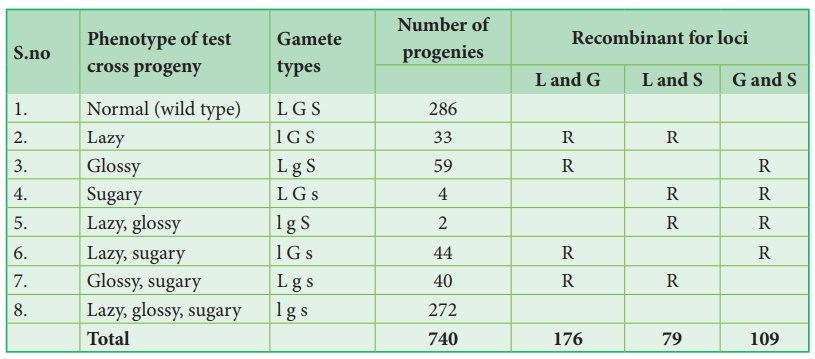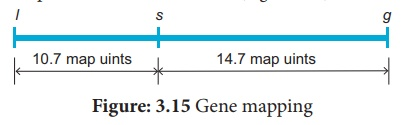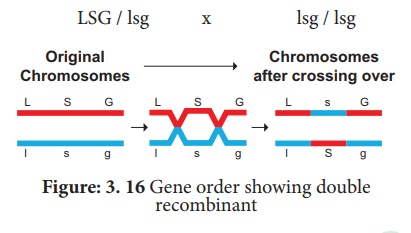Home | | Botany 12th Std | Genetic Mapping

# Genetic Mapping

Genes are present in a linear order along the chromosome.

Genetic Mapping

Genes are present in a linear order along the chromosome. They are present in a specific location called locus (plural: loci). The diagrammatic representation of position of genes and related distances between the adjacent genes is called genetic mapping. It is directly proportional to the frequency of recombination between them. It is also called as linkage map. The concept of gene mapping was first developed by Morgan’s student Alfred H Sturtevant in 1913. It provides clues about where the genes lies on that chromosome.

## Map distance

The unit of distance in a genetic map is called a map unit (m.u). One map unit is equivalent to one percent of crossing over (Figure 4. ). One map unit is also called a centimorgan (cM) in honour of T.H. Morgan. 100 centimorgan is equal to one Morgan (M). For example: A distance between A and B genes is estimated to be 3.5 map units. It is equal to 3.5 centimorgans or 3.5 % or 0.035 recombination frequency between the genes.Genetic maps can be constructed from a series of test crosses for pairs of genes called two point crosses. But this is not efficient because double cross over is missed.

## Three point test cross

A more efficient mapping technique is to construct based on the results of three-point test cross. It refers to analyzing the inheritance patterns of three alleles by test crossing a triple recessive heterozygote with a triple recessive homozygote. It enables to determine the distance between the three alleles and the order in which they are located on the chromosome. Double cross overs can be detected which will provide more accurate map distances.

Three-point test cross can be best understood by considering following an example.

In maize (corn), the three recessive alleles are

1.  l for lazy or prostrate growth habit

2.  g for glossy leaf

3.  s for sugary endosperm

These three recessive alleles (l g s) are crossed with wild type dominant alleles (L G S).Parents       LGS / LGS  x        lgs / lgs

Gametes      LGS  x        lgs

F1 trihybrid          LGS / lgs

Test cross

(Heterozygous F1 crosses with

triple recessive alleles)

LGS / lgs    x        lgs / lgs

This trihybrid test cross produces 8 different types (23=8) of gametes in which 740 progenies are observed. The following table shows the result obtained from a test cross of corn with three linked genes.

## The analysis of a three-point cross:From the above result, we must be careful to observe parental (P) and recombinant (R) types. First note that parental genotypes for the triple homozygotes are L G S and l g s, then analyse two recombinant loci at a time orderly L G/ l g, L S/ l s and G S/ g s. In this any combination other than these two constitutes a recombinant (R).

Let’s analyse the loci of two alleles at a time starting with L and G Since the L G and l g parental genotypes the recombinants will be L g and l G. The Recombinant frequency (RF) for these two alleles can be calculated as followsFor L and S loci, the recombinants are L s and l S. The Recombinant frequency (RF) will be as followsFor G and S loci, the recombinants are G s and g S. The Recombinant frequency (RF) will be as followsAll the loci are linked, because all the RF values are considerably less than 50%. In this L G loci show highest RF value, they must be farthest apart. Therefore, the S locus must lie between them. The order of genes should be l s g. A genetic map can be drawn as follows: (Figure 3.15)A final point note that two smaller map distances, 10.7 m.u and 14.7., is add up to 25.4 m.u., which is greater than 23.7 m.u., the distance calculated for l and g. we must identify the two least number of progenies (totaling 8) in relation to recombination of L and G. These two least progenies are double recombinants arising from double cross over. The two least progenies not only counted once should have counted each of them twice because each represents a double recombinant progeny.

Hence, we can correct the value adding the numbers 33+59+44+40+4+4+2+2=188. Of the total of 740, this number exactly 25.4 %, which is identical with the sum of two component values.

The test cross parental combination can be re written as follows:## Uses of genetic mapping

·         It is used to determine gene order, identify the locus of a gene and calculate the distances between genes.

·         They are useful in predicting results of dihybrid and trihybrid crosses.

·         It allows the geneticists to understand the overall genetic complexity of particular organism.

Tags : Botany : Chromosomal Basis of Inheritance , 12th Botany : Chapter 3 : Chromosomal Basis of Inheritance
Study Material, Lecturing Notes, Assignment, Reference, Wiki description explanation, brief detail
12th Botany : Chapter 3 : Chromosomal Basis of Inheritance : Genetic Mapping | Botany : Chromosomal Basis of Inheritance